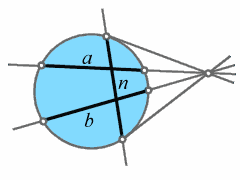# Characterisations of Geometric Transformations

Our aim is to characterise transformation groups via the invariance of a single geometric notion.## Plücker Transformations

This term has been coined by Walter Benz (Hamburg, Germany) in his book Geometrische Transformationen for a group of transformations which preserve a single reflexive and symmetric relation.

For example, let G be a group acting faithfully on the set of lines of a hyperbolic space H such that any two lines that meet orthogonally go over to lines with the same property. Then G is isomorphic (as a transformation group) to the group of hyperbolic motions of H up to the exceptional case when dim H = 3.

In a three-dimensional hyperbolic space the situation is completely different due to the existence of non-continuous automorphisms of the field of complex numbers.

The picture on the left hand side shows two ultraparallel lines a, b in the Cayley-Klein model of the hyperbolic plane together with their common perpendicular line n.

## Publications

• H. Brauner: Über die von Kollineationen projektiver Räume induzierten Geradenabbildungen, Sb. österr. Akad. d. Wiss. 197 (1988), 327-332.
• H. Brauner: Eine Kennzeichung der Ähnlichkeiten affiner Räume mit definiter Orthogonalitätsstruktur, Geom. Dedicata 29 (1989), 45-51.
• H. Havlicek: On Plücker transformations of generalized elliptic spaces, Rend. Mat., Ser. VII, 14 (1995), 39-54.
Preprint (PDF)
• H. Havlicek: On isomorphisms of Grassmann spaces, Mitt. Math. Ges. Hamburg 14 (1995), 117-120.
Preprint (PDF)
• H. Havlicek: Symplectic Plücker transformations, Math. Pannon. 46 (1995), 145-153.
Preprint (PDF)
• H. Havlicek: A characteristic property of elliptic Plücker transformations, J. Geometry 58 (1997), 106-116.
Preprint (PDF)
• H. Havlicek: Isomorphisms of affine Plücker spaces, In: N.K. Artémiadis, N.K. Stephanidis (eds.): Proceedings of the 4th International Conference of Geometry Thessaloniki 1996, Aristoteles University of Thessaloniki 1997, pp.171-178.
Preprint (PDF)
• H. Havlicek: Chow's theorem for linear spaces, Discrete Math. 208/209 (1999), 319-324.
Preprint (PDF)
• K. List: Orthogonalitätstreue Plückertransformationen hyperbolischer Räume, Doctoral Thesis, Vienna University of Technology, 1998.
Full Text (PDF)
• K. List: On orthogonality-Preserving Plücker transformations of hyperbolic spaces, Abh. math. Sem. Univ. Hamburg 70 (2000), 63-75.
Preprint (PDF)
• K. List: Harmonic mappings and hyperbolic Plücker transformations, J. Geometry 70 (2001), 108-116.
Preprint (PDF)
• H. Havlicek, K. List, and C. Zanella: On automorphisms of flag spaces, Linear Multilinear Algebra 50 (2002), 241-251.
Preprint (PDF)
• A. Blunck and H. Havlicek: On bijections that preserve complementarity of subspaces, Discrete Math. 301 (2005), 46-56.
Preprint (PDF)
• H. Havlicek and M. Pankov: Transformations on the product of Grassmann spaces, Demonstratio Math. 38 (2005), 675-688.
Preprint (PDF)
• H. Havlicek and J. Gmainer: Isometries and collineations of the Cayley surface, Innov. Incidence Geom., 2 (2005), 109-127.
Preprint (PDF)

Andrea Blunck
Johannes Gmainer
Hans Havlicek
Klaus List
A Tribute to Heinrich Brauner (1928-1990)
Theory of Linear Mappings
Geometry of Matrices
Sitemap